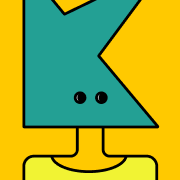# CML- Chapter 5: Integers

\$31880918404805
0 ratings
In elementary school, the integers are typically briefly covered in different grade levels. In grades two through four children may explore what a negative number is, and in grades five and six they perform some basic calculations with integers. Some children are able to use and even invent negative numbers in their self-generated subtraction algorithms. In its discussion of integers, this chapter explores children’s understandings of negative numbers, the varied meanings of the minus sign, the different ways that children might learn addition and subtraction of integers, some possible explanations for multiplying negative and positive numbers, and children’s thinking about negative numbers in the context of problems.
362 KB
14 pages
\$3

0 ratings